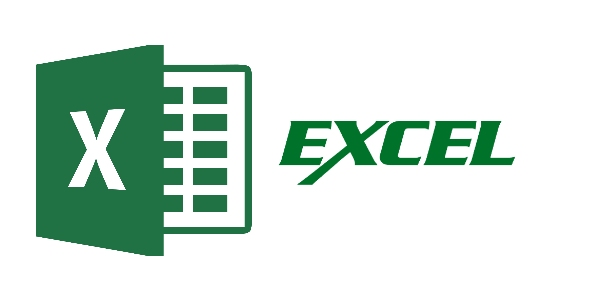# Advanced Excel For Actuaries Quiz

5 Questions | Attempts: 322SettingsSee if you got the correct answers to the assignment.

• 1.
Using the INDEXMATCH method, what formula did you enter into cell D7 to get the Plus brand Camera warranty price?
• 2.
On the Summary tab, what formula did you use to get Region 6's Aug value (cell G9)?
• A.

=INDIRECT("'"&F\$1&"'!"&\$L9)

• B.

=INDIRECT("'"&G\$1&"'!"&\$L2)

• C.

=INDIRECT("'"&G\$1&"'!"&\$L9)

• D.

=SUM(INDIRECT(H1&"!"&"B2:B13"))

• 3.
To create a dynamic range for the last 10 sales values, what does "COUNT(DynamicCharts!\$B\$3:\$B\$1000)-1" in the formula: "=OFFSET(DynamicCharts!\$B\$3,COUNT(DynamicCharts!\$B\$3:\$B\$1000)-1,0,-10,1)"
• A.

It is the new range width

• B.

It represents the number of rows down from the reference point

• C.

It represents the height of the new dynamic range

• D.

It represents the number of columns over from the reference point

• 4.
What Total Warranty Sales for 2015 did you get as an answer?
• A.

12964

• B.

19753

• C.

22975

• D.

27852

• 5.
What is the correct formula to parse the Region number for the first part of the text string cell F2?
• A.

=if(left(F2,2)="10","10",left(F2,1))

• B.

=left(F2,1)

• C.

=if(find(F2,2,2)="10","10",left(F2,2))

• D.

=left(F2,2)

## Related TopicsBack to top
×

Wait!
Here's an interesting quiz for you.